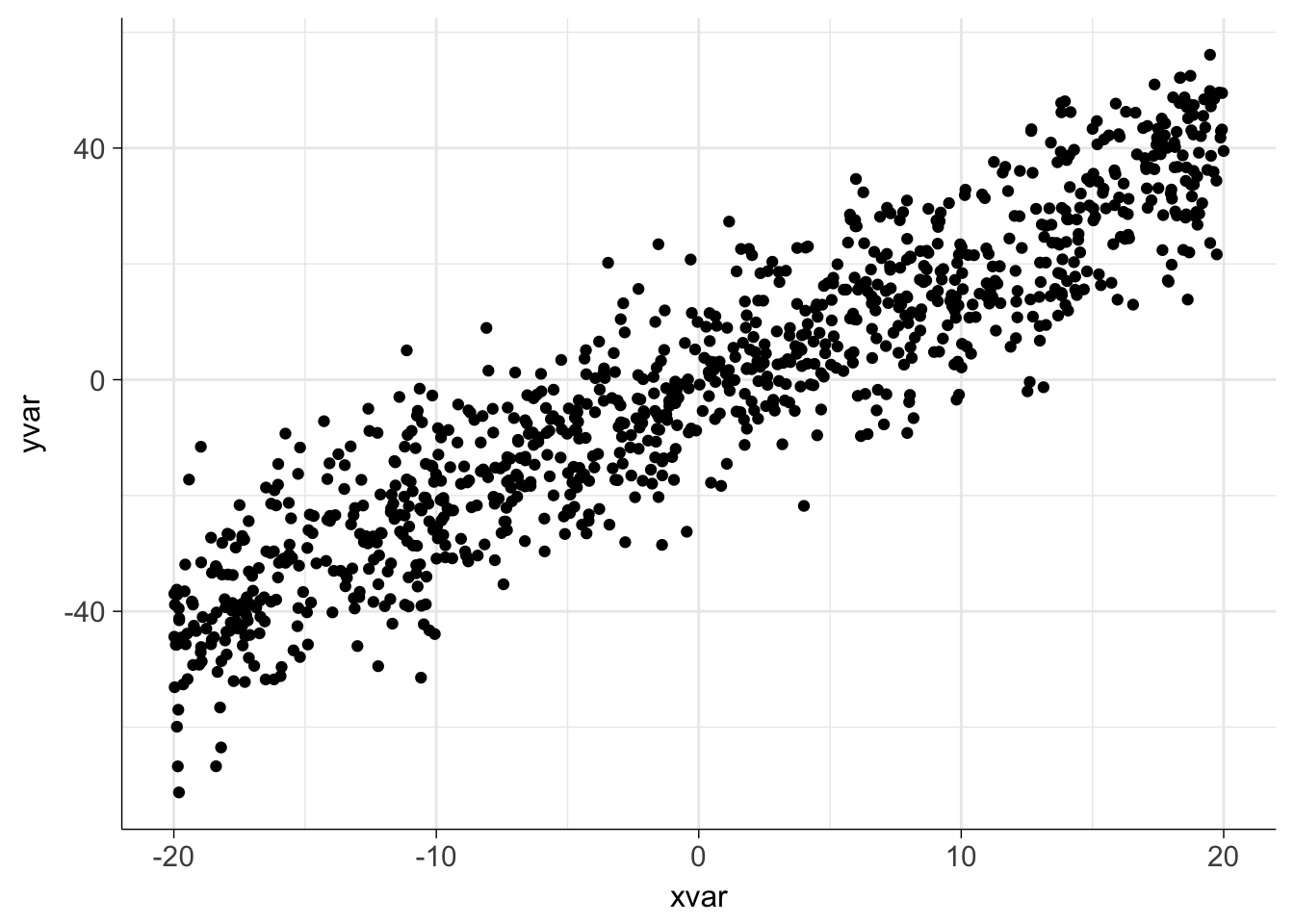This is a demo of the Metropolis-Hastings Markov-Chain Monte Carlo (MH-MCMC) algorithm, coded up with just base R functions (except the plotting stuff). Here it is used to estimate the slope and intercept parameters of a simulated dataset, as one would usually do with an OLS linear regression model. Inline comments explain what is happening at the most crucial steps. Most of the action is in the file `mh-mcmc_from_scratch_functions.r`, but the main function `mh_mcmc()` is reproduced below for reference. Download the `.r` source for this example and the associated functions to play around with the code and try different combos of parameter values!

NOTE: the implementation here is based on the design from this very nice post. For some nice discussion of how the algorithm works, see that post and the ones it links to. The most important differences here are that (i) here we focus on just the slope and intercept parameters, the two that people are most likely to be interested in understanding in practical data analysis scenarios; and (ii) all the functions here are fully encapsulated and don’t make use of global variables (i.e. they are fully portable). This means that it should be quite straightforward to extend the strategy to more complex sets of parameters. That said, you are probably better off using Stan or another modern framework for Bayesian inference – this is mostly just meant for conceptual/educational purposes!

``lefftpack::lazy_setup() # devtools::install_github("lefft/lefftpack")``
``````## >> these packages are now attached:
##      lefftpack reshape2 ggplot2 magrittr dplyr``````
``## >> custom `ggplot2::` plot theme is now set``
``````# or just attach these packages:
# library("dplyr"); library("magrittr"); library("reshape2") library("ggplot2");``````

### Simulate some fake data from pre-determined parameters

``````# functions in this file implement the algorithm for unknown `m` and `b`.
# some have parameters that can be tinkered with for illustrative purposes.
# try playing around with the priors in particular.
source("mh-mcmc_from_scratch_functions.r")

# simulated data whose parameters we will recover with MH-MCMC
b <- -2
m <- 2

n <- 1000
mean_noise <- 0
sd_noise <- 10

xvar <- runif(n, min=-20, max=20)
yvar <- m * xvar + b + rnorm(n=n, mean=mean_noise, sd=sd_noise)

qplot(xvar, yvar)````````lm(yvar ~ xvar)\$coefficients``
``````## (Intercept)        xvar
##   -1.458900    2.041228``````
``````true_b <- as.numeric(lm(yvar ~ xvar)\$coefficients["(Intercept)"])
true_m <- as.numeric(lm(yvar ~ xvar)\$coefficients["xvar"])``````

### Execute the algorithm to recover intercept and slope parameters

``````# `n_iters`-many runs, with start vals of b=m=0 and .25 burn-in rate
start <- c(0, 0)
n_iters <- 2500
burnin_rate <- .25

# execute the algorithm
markov_chain <- mh_mcmc(xvar, yvar, start, n_iters, burnin_rate)

# the ranges of values tried for each parameter are:
range(markov_chain[, 1])``````
``##  -2.583746 -0.499530``
``range(markov_chain[, 2])``
``##  1.937315 2.120304``
``````# note that all params are accepted or rejected in one shot
apply(markov_chain, MARGIN=2, function(col) length(unique(col)))``````
``````## theta_b theta_m
##     380     380``````
``````sim_info <- paste0(
"\nMCMC info: 2.5k iterations; .25 burn-in rate; start values b=0, m=0\n",
"data info: 1k points sampled from f(x) = 2x -2 + N(0, 10), ",
"for x ~ Unif(-20, 20)"
)
# visualize the posteriors and random walk progression for each param
plot_mcmc(chain=markov_chain, true_b=true_b, true_m=true_m, sim_info=sim_info)``````